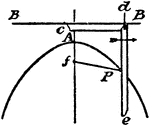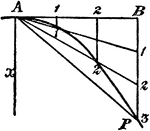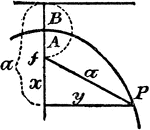### Construction Of A Parabola

An illustration showing how to construct a parabola by plotting. "Having given the axis, vertex, and…### Construction Of A Parabola

An illustration showing how to construct a parabola using a pencil and a string. "Having given the two…### Construction Of A Parabola

An illustration showing how to construct a parabola. "Given the vertex A, axis x, and a point P. Draw…### Construction Of A Parabola

An illustration showing how to construct a parabola. "Given the axis of ordinate B, and vertex A. Take…